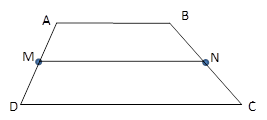Chapter 4.4, Problem 14EElementary Geometry For College St...

7th Edition
Alexander + 2 others
ISBN: 9781337614085

Solutions

Chapter
SectionElementary Geometry For College St...

7th Edition
Alexander + 2 others
ISBN: 9781337614085
Textbook Problem

In Exercises 11 to 16, the drawing shows trapezoid ABCD with A B ¯ | | D C ¯ ; also, M and N are midpoints of A D ¯ and B C ¯ , respectively.Exercises 11-16Given: A B = 7 x + 5 , D C = 4 x − 2 and M N = 5 x + 3 Find: x .

To determine

To Find:

The value of x.

Explanation

Consider the following trapezoid ABCD.

Given:

AB=7x+5, DC=4x2 and MN=5x+3.

Also in the given trapezoid, AB¯||DC¯.

M is the midpoint of the side AD¯ and N is the midpoint of the side BC¯.

MN is the median of the trapezoid ABCD.

We know that the length of the median (m) of a trapezoid is the average of the lengths of the bases

Still sussing out bartleby?

Check out a sample textbook solution.

See a sample solution

The Solution to Your Study Problems

Bartleby provides explanations to thousands of textbook problems written by our experts, many with advanced degrees!

Get Started

Are the following graphs of functions? Explain. a. b. c. d.

Applied Calculus for the Managerial, Life, and Social Sciences: A Brief Approach

Find the mean for the following set of scores: 2, 7, 9, 4, 5, 3, 0, 6

Essentials of Statistics for The Behavioral Sciences (MindTap Course List)

Evaluate the definite integral. 12e1/xx2dx

Single Variable Calculus: Early Transcendentals, Volume I

The solution to y = y2 with y(1)=13 is: a) y=1x+2 b) y=lnx+13 c) y=12x216 d) y=12x16

Study Guide for Stewart's Single Variable Calculus: Early Transcendentals, 8th

The vector represented by where A(4, 8) and B(6, 6)is:

Study Guide for Stewart's Multivariable Calculus, 8th